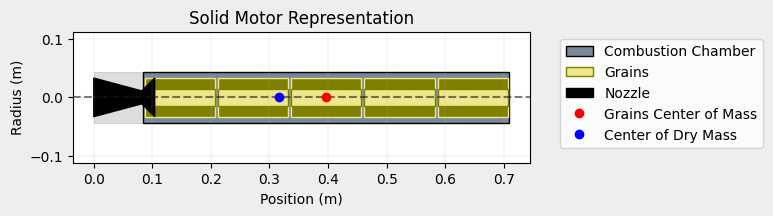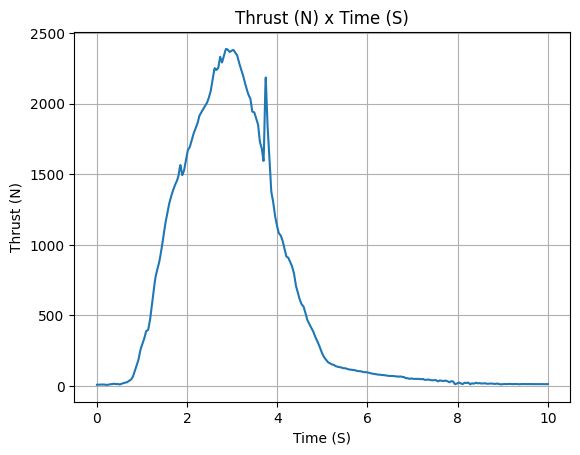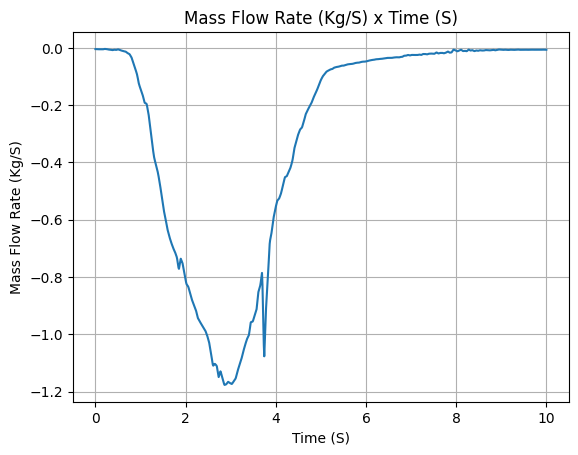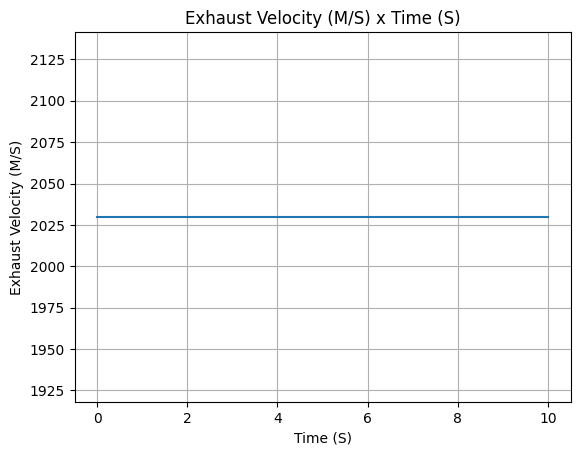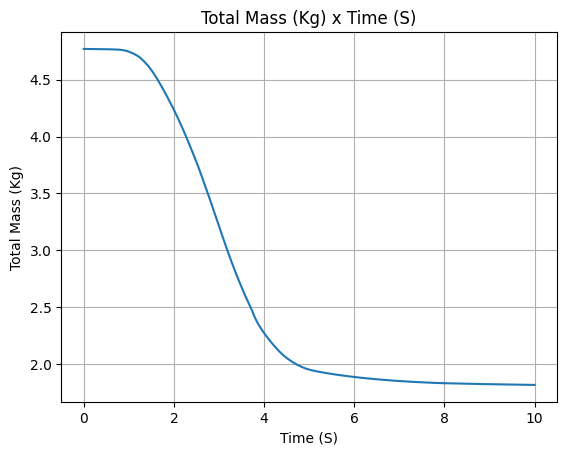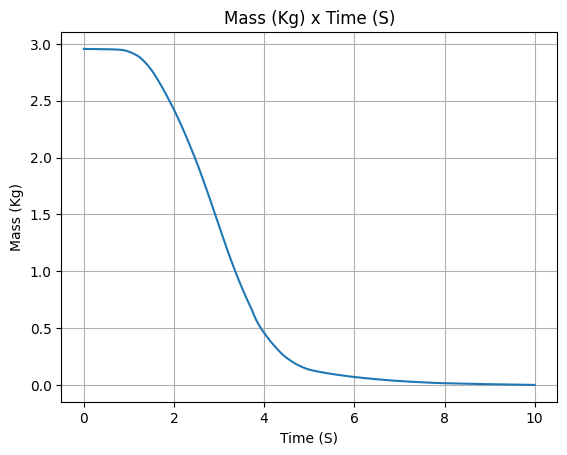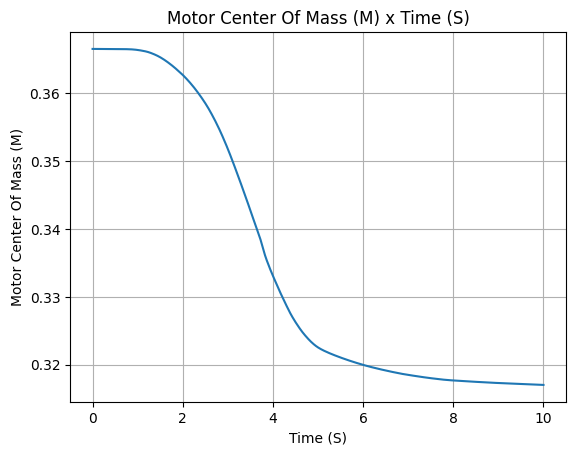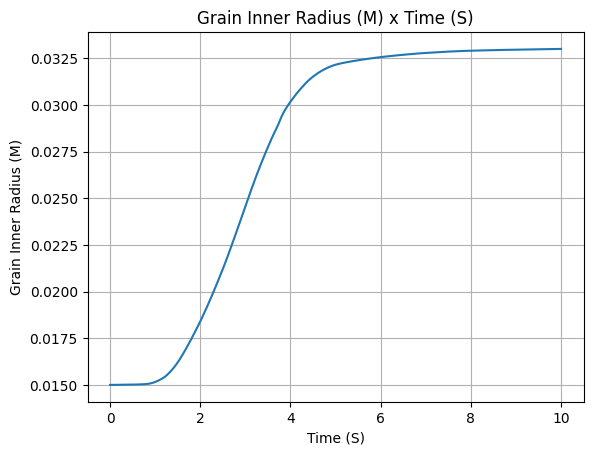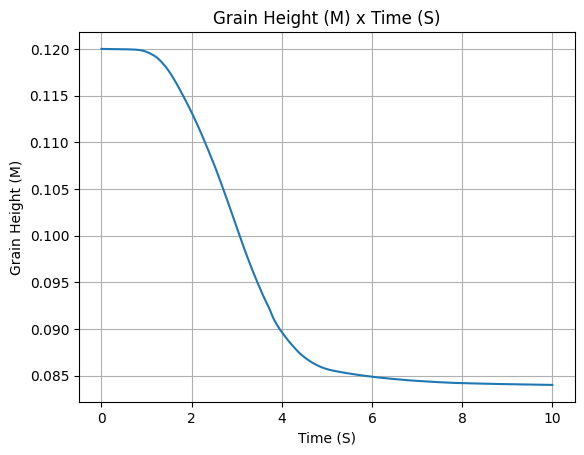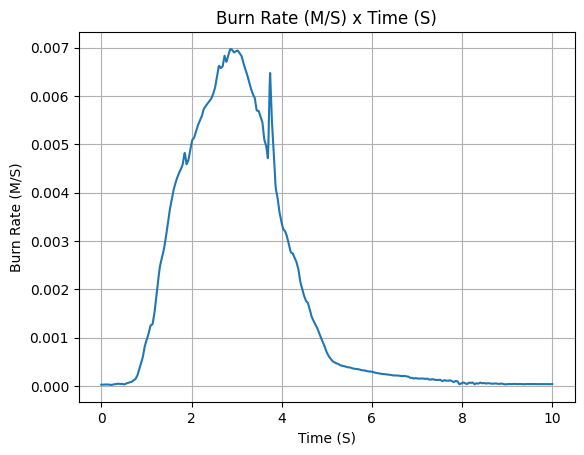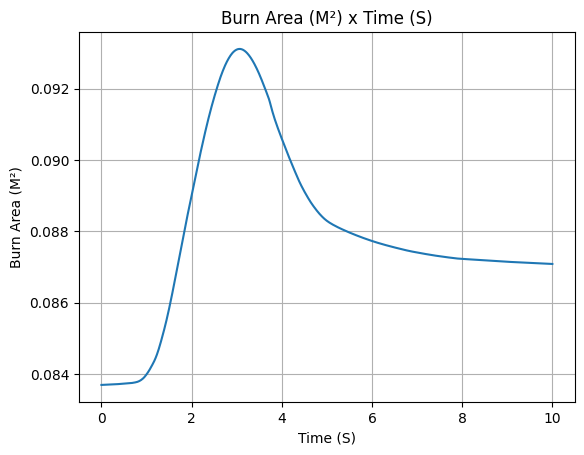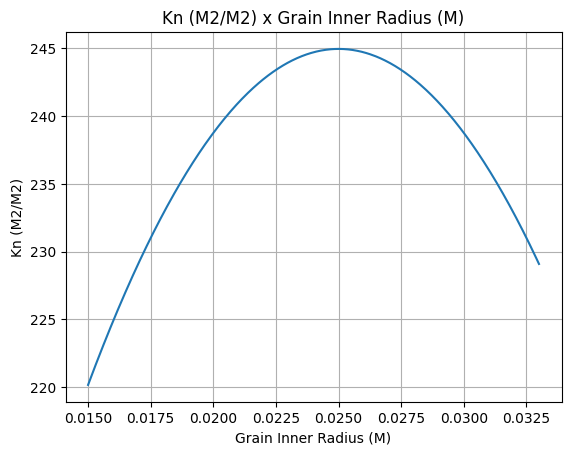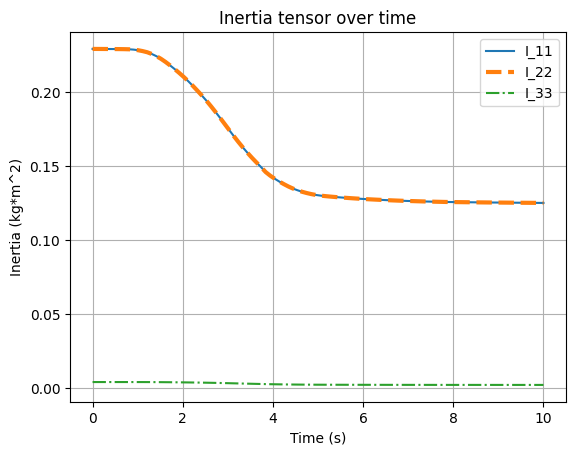# Thrust Source#

The thrust source is of most importance when analyzing several trajectory attributes, including the out of rail velocity, thrust to weight ratio, apogee and many others. Let’s create a new motor and take a closer look at this functionality.

## Constant Thrust#

When passing an `int` or `float`, the thrust will be considered constant in time.

```from rocketpy import SolidMotor

solid_constant = SolidMotor(
thrust_source=1500,
dry_mass=1.815,
dry_inertia=(0.125, 0.125, 0.002),
center_of_dry_mass_position=0.317,
grains_center_of_mass_position=0.397,
burn_time=3.9,
grain_number=5,
grain_separation=5 / 1000,
grain_density=1815,
grain_initial_height=120 / 1000,
interpolation_method="linear",
nozzle_position=0,
coordinate_system_orientation="nozzle_to_combustion_chamber",
)
```

Let’s call the `info()` method to see what kind of information we get. Alternatively, we could use the `all_info()` method to get a more complete output.

```solid_constant.info()
```
```Nozzle Details

Grain Details
Number of Grains: 5
Grain Spacing: 0.005 m
Grain Density: 1815 kg/m3
Grain Height: 0.12 m
Grain Volume: 0.000 m3
Grain Mass: 0.591 kg

Motor Details
Total Burning Time: 3.9 s
Total Propellant Mass: 2.956 kg
Average Propellant Exhaust Velocity: 1979.085 m/s
Average Thrust: 1500.000 N
Maximum Thrust: 1500.0 N at 0.0 s after ignition.
Total Impulse: 5850.000 Ns

```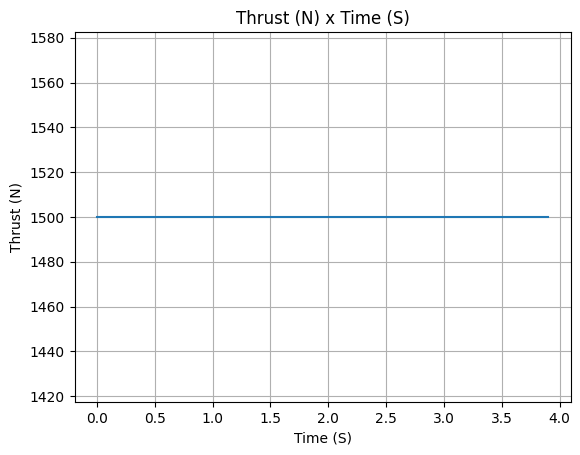## Thrust From Static Firings (CSV Files)#

Usually one has much more precise information about the motor and wishes to specify a directory containing a .csv file.

Note

The first column of the .csv file must be time in seconds and the second column must be thrust in Newtons. The file must contain no headers.

That can be done as follows:

```solid_csv = SolidMotor(
thrust_source="../data/motors/keron/thrustCurve.csv",
dry_mass=1.815,
dry_inertia=(0.125, 0.125, 0.002),
center_of_dry_mass_position=0.317,
grains_center_of_mass_position=0.397,
burn_time=3.9,
grain_number=5,
grain_separation=5 / 1000,
grain_density=1815,
grain_initial_height=120 / 1000,
interpolation_method="linear",
nozzle_position=0,
coordinate_system_orientation="nozzle_to_combustion_chamber",
)
solid_csv.info()
```
```Nozzle Details

Grain Details
Number of Grains: 5
Grain Spacing: 0.005 m
Grain Density: 1815 kg/m3
Grain Height: 0.12 m
Grain Volume: 0.000 m3
Grain Mass: 0.591 kg

Motor Details
Total Burning Time: 3.9 s
Total Propellant Mass: 2.956 kg
Average Propellant Exhaust Velocity: 474.704 m/s
Average Thrust: 359.790 N
Maximum Thrust: 1068.36 N at 1.508 s after ignition.
Total Impulse: 1403.183 Ns

```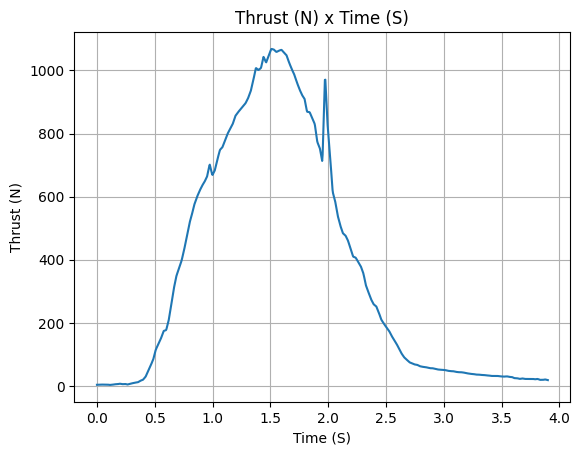Attention

Beware of noisy data. RocketPy will not filter the data for you.

The thrust curve will be integrated to obtain the total impulse. This can lead to a significant error in the total impulse if the data is too noisy.

Also one is able to specify a certain interpolation method. That can be done by simply changing the `interpolation_method` parameter to `spline` , `akima` or `linear`. Default is set to `linear`.

## Eng Files (RASP)#

Most rocket motors providers share the thrust curve from their motors using the RASP file format (`.eng` files). RocketPy can import such files as the thrust source.

Note

If you have a thrust curve in a `.csv` file, RocketPy can also read your data and exported as a `.eng` file. This can be done by using the `rocketpy.Motor.export_eng()` Motor method.

```solid_eng = SolidMotor(
thrust_source="../data/motors/Cesaroni_M1670.eng",
dry_mass=1.815,
dry_inertia=(0.125, 0.125, 0.002),
center_of_dry_mass_position=0.317,
grains_center_of_mass_position=0.397,
burn_time=3.9,
grain_number=5,
grain_separation=5 / 1000,
grain_density=1815,
grain_initial_height=120 / 1000,
interpolation_method="linear",
nozzle_position=0,
coordinate_system_orientation="nozzle_to_combustion_chamber",
)
```

This time we want to try the all_info() to capture more details about the motor.

```solid_eng.all_info()
```
```Nozzle Details

Grain Details
Number of Grains: 5
Grain Spacing: 0.005 m
Grain Density: 1815 kg/m3
Grain Height: 0.12 m
Grain Volume: 0.000 m3
Grain Mass: 0.591 kg

Motor Details
Total Burning Time: 3.9 s
Total Propellant Mass: 2.956 kg
Average Propellant Exhaust Velocity: 2038.745 m/s
Average Thrust: 1545.218 N
Maximum Thrust: 2200.0 N at 0.15 s after ignition.
Total Impulse: 6026.350 Ns

```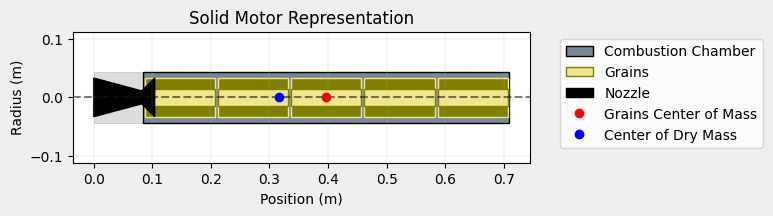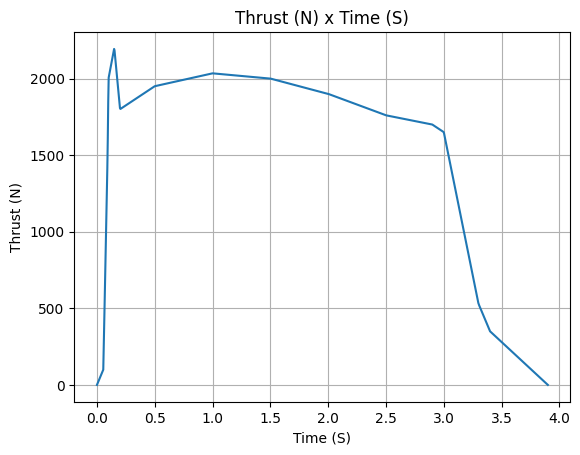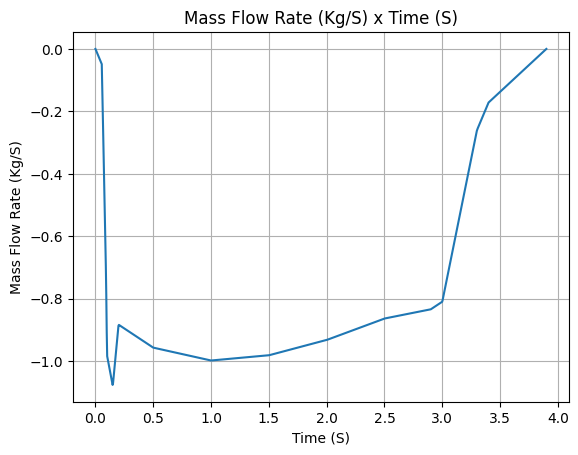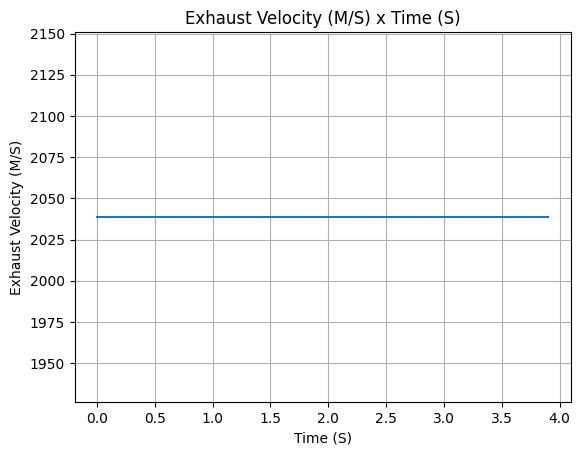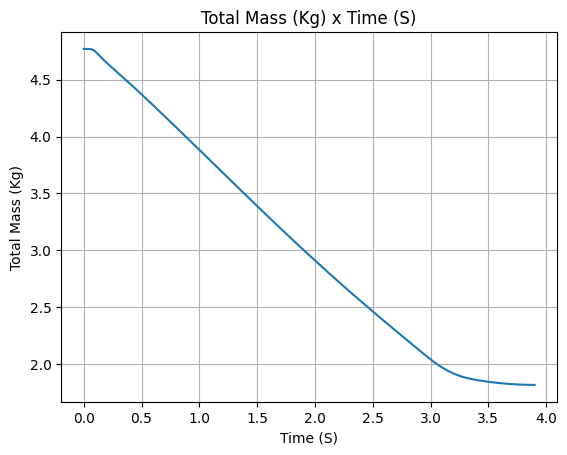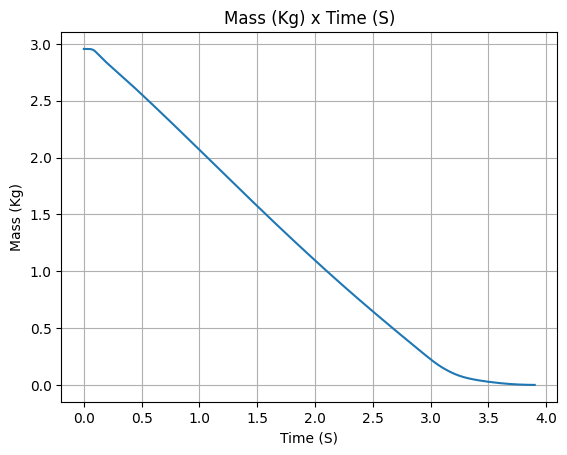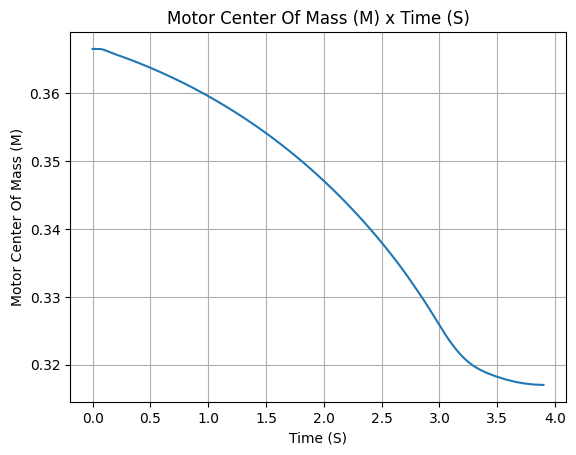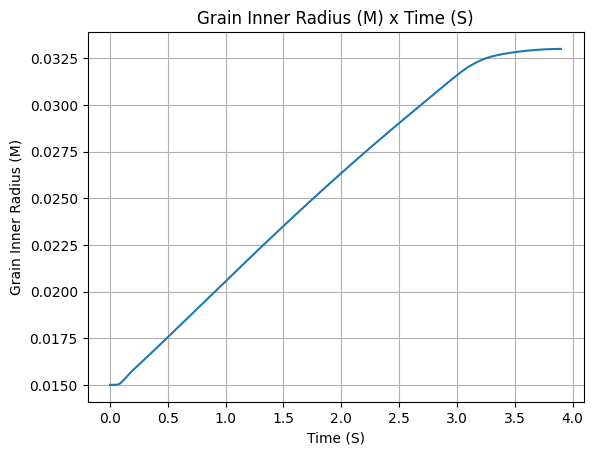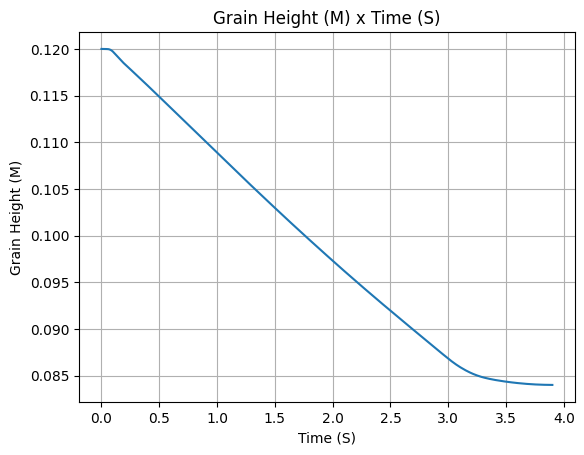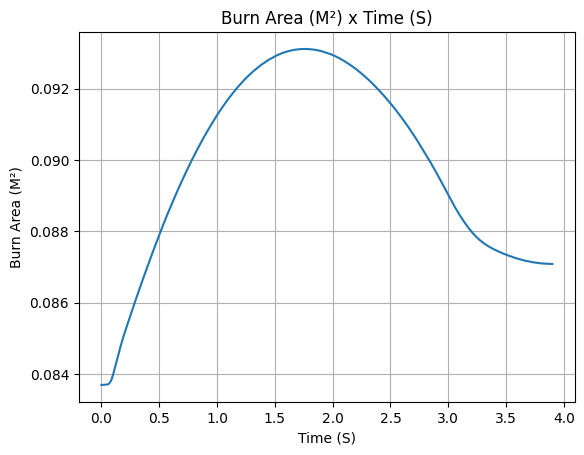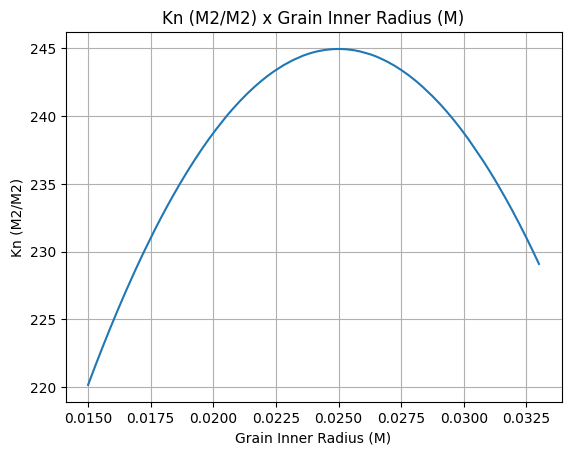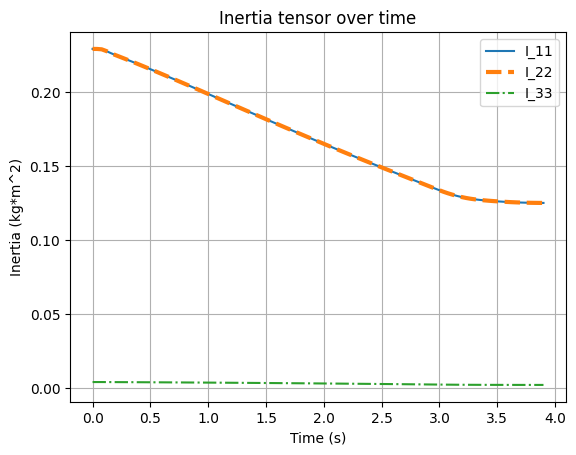## Lambda Functions#

There is also a fourth option where one specifies the thrust source parameter by passing a callable function like below.

Lambda functions are particularly useful in Python, and therefore the SolidMotor class also supports them. Let’s see how to use it.

```solid_lambda = SolidMotor(
thrust_source=lambda x: 1 / (x + 1),
dry_mass=1.815,
dry_inertia=(0.125, 0.125, 0.002),
center_of_dry_mass_position=0.317,
grains_center_of_mass_position=0.397,
burn_time=3.9,
grain_number=5,
grain_separation=5 / 1000,
grain_density=1815,
grain_initial_height=120 / 1000,
interpolation_method="linear",
nozzle_position=0,
coordinate_system_orientation="nozzle_to_combustion_chamber",
)
solid_lambda.info()
```
```Nozzle Details

Grain Details
Number of Grains: 5
Grain Spacing: 0.005 m
Grain Density: 1815 kg/m3
Grain Height: 0.12 m
Grain Volume: 0.000 m3
Grain Mass: 0.591 kg

Motor Details
Total Burning Time: 3.9 s
Total Propellant Mass: 2.956 kg
Average Propellant Exhaust Velocity: 0.538 m/s
Average Thrust: 0.408 N
Maximum Thrust: 1.0 N at 0.0 s after ignition.
Total Impulse: 1.590 Ns

```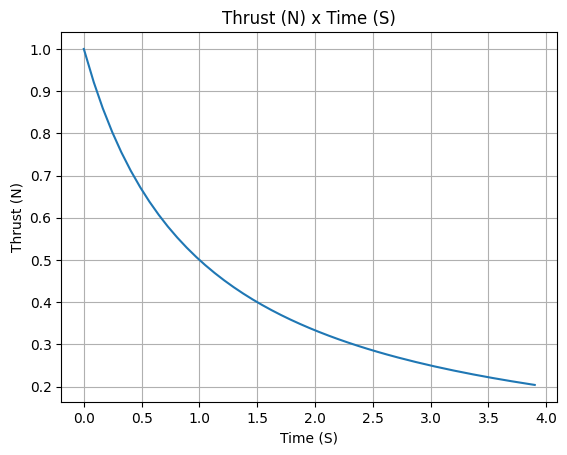## Reshaping and interpolating the thrust curve#

RocketPy can rescale a given curve to match new specifications when impulse and burn out time are expected to vary only slightly. That can be done by passing the `reshape_thrust_curve` parameter as a list of two elements. The first element is the new burn out time in seconds and the second element is the new total impulse in Ns.

Here we will reshape the thrust curve by setting the new burn out time in seconds to 10 and the new total impulse to be 6000 Ns.

```solid_reshaped = SolidMotor(
thrust_source="../data/motors/keron/thrustCurve.csv",
dry_mass=1.815,
dry_inertia=(0.125, 0.125, 0.002),
reshape_thrust_curve=[10, 6000],
center_of_dry_mass_position=0.317,
grains_center_of_mass_position=0.397,
burn_time=3.9,
grain_number=5,
grain_separation=5 / 1000,
grain_density=1815,
grain_initial_height=120 / 1000,
interpolation_method="linear",
nozzle_position=0,
coordinate_system_orientation="nozzle_to_combustion_chamber",
)
```

Pay close attention to the newly generated curve and be aware of the changes the rescale has produced regarding the physical quantities.

```solid_reshaped.all_info()
```
```Nozzle Details

Grain Details
Number of Grains: 5
Grain Spacing: 0.005 m
Grain Density: 1815 kg/m3
Grain Height: 0.12 m
Grain Volume: 0.000 m3
Grain Mass: 0.591 kg

Motor Details
Total Burning Time: 10.0 s
Total Propellant Mass: 2.956 kg
Average Propellant Exhaust Velocity: 2029.830 m/s
Average Thrust: 600.000 N
Maximum Thrust: 2388.940104826747 N at 2.8593098217671598 s after ignition.
Total Impulse: 6000.000 Ns

```